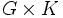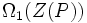# Normal-isomorph-free not implies isomorph-free in finite

This article gives the statement and possibly, proof, of a non-implication relation between two subgroup properties. That is, it states that every subgroup satisfying the first subgroup property (i.e., normal-isomorph-free subgroup) need not satisfy the second subgroup property (i.e., isomorph-free subgroup)
View a complete list of subgroup property non-implications | View a complete list of subgroup property implications
EXPLORE EXAMPLES YOURSELF: View examples of subgroups satisfying property normal-isomorph-free subgroup but not isomorph-free subgroup|View examples of subgroups satisfying property normal-isomorph-free subgroup and isomorph-free subgroup

## Statement

### Verbal statement

We may have a group with a normal subgroup such that there is no other normal subgroup isomorphic to it, but there are other non-normal subgroups isomorphic to it.

## Proof

### An example involving the symmetric group

Further information: symmetric group:S3

Let$G$ be the symmetric group on three letters and$K$ be the cyclic group of order two.

•$1 \times K$ is a normal subgroup of$G \times K$. Further, if$L$ is cyclic of order two and normal in$G \times K$, then the projection of$L$ in$G$ is normal in$G$. Since$G$ has no normal subgroups of order two, the projection of$L$ in$G$ is trivial, so$L = 1 \times K$. Thus,$1 \times K$ is a normal-isomorph-free subgroup of$G$.
• On the other hand,$1 \times K$ is not isomorph-free in$G \times K$: We can find a two-element subgroup$H$ of$G$, and we then have$H \times 1 \cong 1 \times K$.

### An example involving the dihedral group

Further information: dihedral group:D8

Let$G$ be the dihedral group of order eight:$G = \langle a,x \mid a^4 = x^2 = e, xax^{-1} = a^{-1} \rangle$.

Let$H$ be the center of$G$, so$H = \langle a^2 \rangle$. Then:

•$H$ is normal-isomorph-free: It is the only normal subgroup of order two.
•$H$ is not isomorph-free: There are other subgroups of order two, such as$\langle x \rangle$.

### A generic example

For a group of prime power order, the following is true: if the center is of prime order, it is normal-isomorph-free. This follows from the fact that nilpotent implies center is normality-large: in a nilpotent group, the intersection between the center and any nontrivial normal subgroup is nontrivial. On the other hand, the center is rarely isomorph-free (an example where it is isomorph-free is the generalized quaternion group).

More generally, if$P$ is a$p$-group and$\Omega_1(Z(P))$ denotes the set of elements of order$p$, then if$\Omega_1(Z(P))$ is cyclic of order$p$, it is normal-isomorph-free.